Note: This is an archvied version of our old webpage. Some links might be broken. The current one can be found here.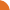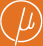Chair for Foundations of Software Reliability and Theoretical Computer Science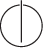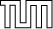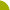Publications - Distinguishing Hidden Markov ChainsReference:

Stefan Kiefer and A. Prasad Sistla. Distinguishing hidden Markov chains. In Proceedings of the 31st Annual Symposium on Logic in Computer Science (LICS), pages 66–75, New York, USA, 2016. ACM.

Abstract:

Hidden Markov Chains (HMCs) are commonly used mathematical models of probabilistic systems. They are employed in various fields such as speech recognition, signal processing, and biological sequence analysis. Motivated by applications in stochastic runtime verification, we consider the problem of distinguishing two given HMCs based on a single observation sequence that one of the HMCs generates. More precisely, given two HMCs and an observation sequence, a distinguishing algorithm is expected to identify the HMC that generates the observation sequence. Two HMCs are called distinguishable if for every epsilon > 0 there is a distinguishing algorithm whose error probability is less than epsilon. We show that one can decide in polynomial time whether two HMCs are distinguishable. Further, we present and analyze two distinguishing algorithms for distinguishable HMCs. The first algorithm makes a decision after processing a fixed number of observations, and it exhibits two-sided error. The second algorithm processes an unbounded number of observations, but the algorithm has only one-sided error. The error probability, for both algorithms, decays exponentially with the number of processed observations. We also provide an algorithm for distinguishing multiple HMCs.

Suggested BibTeX entry:

@inproceedings{16KS-LICS,PDF (376 kB)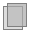SlidesTech report version